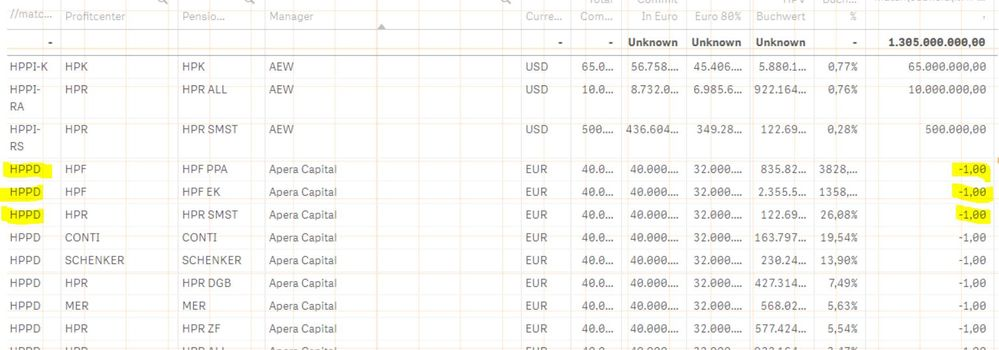# New to Qlik Sense

Discussion board where members can get started with Qlik Sense.

Announcements
Coming your way, the Qlik Data Revolution Virtual Summit. October 27-29. REGISTER
cancel
Showing results for
Did you mean:
HighlightedCreator III

## Problem with if loop with multiple condition

I want to implement  a logic that if  %HPP_Summary_List contains  'HPPD' then if the field  Pensionseinrichtung has a value  'CONTI' it will calculate  [Total Commit] *0.2 , etc  ELSE if the  %HPP_Summary_List does not contain HPPD but other values, it will just return the value of Ttoal commit

Script:

if(
match(subfield(%HPP_Summary_List,'_',1),'HPPD') ,

(
If(Match([Pensionseinrichtung], 'HPK'),Sum(TOTAL {\$<Pensionseinrichtung = {'HPR ALL'}>} [Total Commit] *0.63)) or
If(Match([Pensionseinrichtung], 'HPR ALL'),Sum(TOTAL {\$<Pensionseinrichtung = {'HPR ALL'}>} [Total Commit] *0.14)) or
If(Match([Pensionseinrichtung], 'HPR DGB'),Sum(TOTAL {\$<Pensionseinrichtung = {'HPR DGB'}>} [Total Commit] *0.07)) or
If(Match([Pensionseinrichtung], 'HPR SMST'),Sum(TOTAL {\$<Pensionseinrichtung = {'HPR SMST'}>} [Total Commit] *0.02)) or
If(Match([Pensionseinrichtung], 'HPR ZF'),Sum(TOTAL {\$<Pensionseinrichtung = {'HPR ZF'}>} [Total Commit] *0.06)) or
If(Match([Pensionseinrichtung], 'SCHENKER'),Sum(TOTAL {\$<Pensionseinrichtung = {'SCHENKER'}>} [Total Commit] *0.05)) or
If(Match([Pensionseinrichtung], 'CONTI'),Sum(TOTAL {\$<Pensionseinrichtung = {'CONTI'}>} [Total Commit] *0.02)) or
If(Match([Pensionseinrichtung], 'HPF PPA'),Sum(TOTAL {\$<Pensionseinrichtung = {'HPF PPA'}>} [Total Commit] *0.0003)) or
If(Match([Pensionseinrichtung], 'HPF EK'),Sum(TOTAL {\$<Pensionseinrichtung = {'HPF EK'}>} [Total Commit] *0.0008)) or
If(Match([Pensionseinrichtung], 'MER'),Sum(TOTAL {\$<Pensionseinrichtung = {'MER'}>} [Total Commit] *0.99))),
[Total Commit] )

The script is not generating the desired output.1 Solution

Accepted Solutions
HighlightedCreator III
1.if(match(subfield(%HPP_Summary_List,'_',1),'HPPD'), [Euro 80% HPPD],[Euro 80%])
2. [Euro 80% HPPD] = if(match(subfield(%HPP_Summary_List,'_',1),'HPPD'),
If(Match([Pensionseinrichtung], 'MER'),Sum( [Total Commit] *0.0099*.08),
If(Match([Pensionseinrichtung], 'HPK'),Sum( [Total Commit] *0.63*.08),
If(Match([Pensionseinrichtung], 'HPR ALL'),Sum( [Total Commit] *0.14*.08),
If(Match([Pensionseinrichtung], 'HPR DGB'),Sum( [Total Commit] *0.07*.08),
If(Match([Pensionseinrichtung], 'HPR SMST'),Sum( [Total Commit] *0.02*.08),
If(Match([Pensionseinrichtung], 'HPR ZF'),Sum( [Total Commit] *0.06*.08),
If(Match([Pensionseinrichtung], 'SCHENKER'),Sum( [Total Commit] *0.05*.08),
If(Match([Pensionseinrichtung], 'CONTI'),Sum( [Total Commit] *0.02*.08),
If(Match([Pensionseinrichtung], 'HPF PPA'),Sum( [Total Commit] *0.0003*.08),
If(Match([Pensionseinrichtung], 'HPF EK'),Sum( [Total Commit] *0.0008*.08),
//if(not match(subfield(%HPP_Summary_List,'_',1),'HPPD'),
sum([Total Commit]*.08)
)))))))))))

Solved it myself
2 Replies
HighlightedSpecialist III

if(
%HPP_Summary_List  like '%HPPD%'

try above

---is it working..?

([Pensionseinrichtung], 'HPK'),Sum(TOTAL {\$<Pensionseinrichtung = {'HPR ALL'}>} [Total Commit] *0.63)

Channa
HighlightedCreator III
1.if(match(subfield(%HPP_Summary_List,'_',1),'HPPD'), [Euro 80% HPPD],[Euro 80%])
2. [Euro 80% HPPD] = if(match(subfield(%HPP_Summary_List,'_',1),'HPPD'),
If(Match([Pensionseinrichtung], 'MER'),Sum( [Total Commit] *0.0099*.08),
If(Match([Pensionseinrichtung], 'HPK'),Sum( [Total Commit] *0.63*.08),
If(Match([Pensionseinrichtung], 'HPR ALL'),Sum( [Total Commit] *0.14*.08),
If(Match([Pensionseinrichtung], 'HPR DGB'),Sum( [Total Commit] *0.07*.08),
If(Match([Pensionseinrichtung], 'HPR SMST'),Sum( [Total Commit] *0.02*.08),
If(Match([Pensionseinrichtung], 'HPR ZF'),Sum( [Total Commit] *0.06*.08),
If(Match([Pensionseinrichtung], 'SCHENKER'),Sum( [Total Commit] *0.05*.08),
If(Match([Pensionseinrichtung], 'CONTI'),Sum( [Total Commit] *0.02*.08),
If(Match([Pensionseinrichtung], 'HPF PPA'),Sum( [Total Commit] *0.0003*.08),
If(Match([Pensionseinrichtung], 'HPF EK'),Sum( [Total Commit] *0.0008*.08),
//if(not match(subfield(%HPP_Summary_List,'_',1),'HPPD'),
sum([Total Commit]*.08)
)))))))))))

Solved it myself